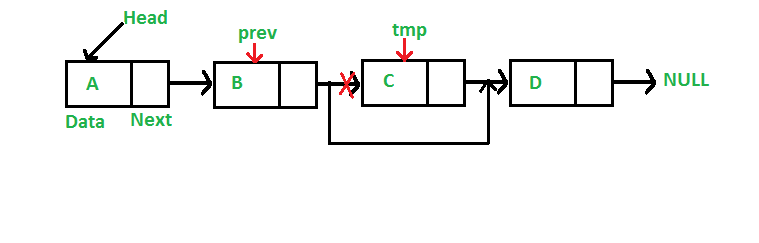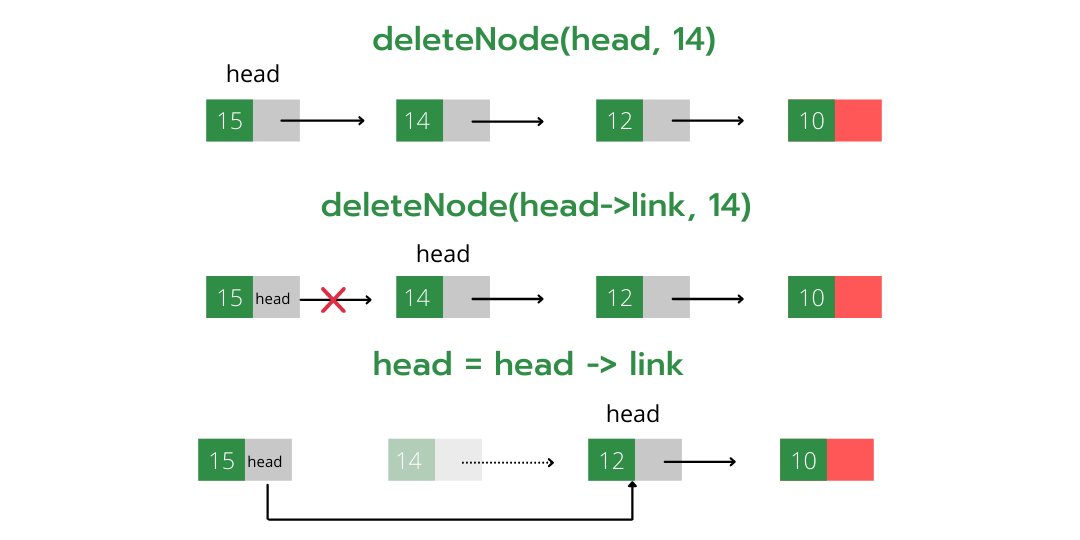# C++ Program for Deleting a Node in a Linked List

• Last Updated : 26 Dec, 2021

We have discussed Linked List Introduction and Linked List Insertion in previous posts on a singly linked list.
Let us formulate the problem statement to understand the deletion process. Given a ‘key’, delete the first occurrence of this key in the linked list

Iterative Method:
To delete a node from the linked list, we need to do the following steps.
1) Find the previous node of the node to be deleted.
2) Change the next of the previous node.
3) Free memory for the node to be deleted.Since every node of the linked list is dynamically allocated using malloc() in C, we need to call free() for freeing memory allocated for the node to be deleted.

## C++

 `// A complete working C++ program to``// demonstrate deletion in singly ``// linked list with class ``#include ``using` `namespace` `std;`` ` `// A linked list node``class` `Node{``public``:``    ``int` `data;``    ``Node* next;``};`` ` `// Given a reference (pointer to pointer)``// to the head of a list and an int, ``// inserts a new node on the front of the``// list. ``void` `push(Node** head_ref, ``int` `new_data)``{``    ``Node* new_node = ``new` `Node();``    ``new_node->data = new_data;``    ``new_node->next = (*head_ref);``    ``(*head_ref) = new_node;``}`` ` `// Given a reference (pointer to pointer)``// to the head of a list and a key, deletes``// the first occurrence of key in linked list ``void` `deleteNode(Node** head_ref, ``int` `key)``{``     ` `    ``// Store head node``    ``Node* temp = *head_ref;``    ``Node* prev = NULL;``     ` `    ``// If head node itself holds``    ``// the key to be deleted``    ``if` `(temp != NULL && temp->data == key)``    ``{``        ``*head_ref = temp->next; ``// Changed head``        ``delete` `temp;            ``// free old head``        ``return``;``    ``}`` ` `    ``// Else Search for the key to be deleted, ``    ``// keep track of the previous node as we``    ``// need to change 'prev->next' */``      ``else``    ``{``    ``while` `(temp != NULL && temp->data != key)``    ``{``        ``prev = temp;``        ``temp = temp->next;``    ``}`` ` `    ``// If key was not present in linked list``    ``if` `(temp == NULL)``        ``return``;`` ` `    ``// Unlink the node from linked list``    ``prev->next = temp->next;`` ` `    ``// Free memory``    ``delete` `temp;``    ``}``}`` ` `// This function prints contents of``// linked list starting from the ``// given node ``void` `printList(Node* node)``{``    ``while` `(node != NULL) ``    ``{``        ``cout << node->data << ``" "``;``        ``node = node->next;``    ``}``}`` ` `// Driver code``int` `main()``{``     ` `    ``// Start with the empty list ``    ``Node* head = NULL;`` ` `    ``// Add elements in linked list``    ``push(&head, 7);``    ``push(&head, 1);``    ``push(&head, 3);``    ``push(&head, 2);`` ` `    ``puts``(``"Created Linked List: "``);``    ``printList(head);`` ` `    ``deleteNode(&head, 1);``    ``puts``("``Linked List after Deletion of 1: ");``     ` `    ``printList(head);``     ` `    ``return` `0;``}`` ` `// This code is contributed by ac121102`

Output:
```Created Linked List:
2  3  1  7
Linked List after Deletion of 1:
2  3  7```

Recursive Method:

To delete a node of a linked list recursively we need to do the following steps.

1.We pass node* (node pointer) as a reference to the function (as in node* &head)

2.Now since the current node pointer is derived from the previous node’s next (which is passed by reference) so now if the value of the current node pointer is changed, the previous next node’s value also gets changed which is the required operation while deleting a node (i.e points previous node’s next to current node’s (containing key) next).

3.Find the node containing the given value.

4.Store this node to deallocate it later using free() function.

5.Change this node pointer so that it points to its next and by performing this previous node’s next also get properly linked.Below is the implementation of the above approach.

## C++

 `// C++ program to delete a node in``// singly linked list recursively`` ` `#include ``using` `namespace` `std;`` ` `struct` `node {``    ``int` `info;``    ``node* link = NULL;``    ``node() {}``    ``node(``int` `a)``        ``: info(a)``    ``{``    ``}``};`` ` `/*``Deletes the node containing 'info' part as val and``alter the head of the linked list (recursive method)``*/``void` `deleteNode(node*& head, ``int` `val)``{``     ` `    ``// Check if list is empty or we ``    ``// reach at the end of the``    ``// list.``    ``if` `(head == NULL) {``        ``cout << "Element not present in the list``";``        ``return``;``    ``}``    ``// If current node is the node to be deleted``    ``if` `(head->info == val) {``        ``node* t = head;``        ``head = head->link; ``// If it's start of the node head``                           ``// node points to second node``        ``delete` `(t); ``// Else changes previous node's link to``                    ``// current node's link``        ``return``;``    ``}``    ``deleteNode(head->link, val);``}`` ` `// Utility function to add a ``// node in the linked list``// Here we are passing head by ``// reference thus no need to``// return it to the main function``void` `push(node*& head, ``int` `data)``{``    ``node* newNode = ``new` `node(data);``    ``newNode->link = head;``    ``head = newNode;``}`` ` `// Utility function to print ``// the linked list (recursive``// method)``void` `print(node* head)``{``     ` `    ``// cout<info << ``' '``;``    ``print(head->link);``}`` ` `int` `main()``{``    ``// Starting with an empty linked list``    ``node* head = NULL;`` ` `    ``// Adds new element at the ``    ``// beginning of the list``    ``push(head, 10);``    ``push(head, 12);``    ``push(head, 14);``    ``push(head, 15);`` ` `    ``// original list``    ``print(head);`` ` `    ``deleteNode(head, 20); ``// Call to delete function``    ``print(head); ``// 20 is not present thus no change in the``                 ``// list`` ` `    ``deleteNode(head, 10);``    ``print(head);`` ` `    ``deleteNode(head, 14);``    ``print(head);`` ` `    ``return` `0;``}`

Output:
```Element not present in the list
15 14 12 10
15 14 12
15 12 ```

Please refer complete article on Linked List | Set 3 (Deleting a node) for more details!

My Personal Notes arrow_drop_up